Next:Entropy-Type MeasuresUp:Continuous Relative Information

Minimum Relative Information Principle

In Chapter 1, we saw that the "principle of maximum entropy" is applicable in situations where information becomes available in the form of expected value or constraints and we wish to determine the underlying probability density. This restriction on the form of available information limits the type of problem which we can consider; the principle being applicable only if the partial information is in the form of averages.

First, as example, consider a situation where we have partial information in the form of averages and also that the prior estimate of the probability distribution is say the Poisson distribution with a certain value of the parameter which is the mean. This problem cannot be handled by M.E.P. If we were to apply the M.E.P. we would not be able to use the partial information which is coded in the estimate of the unknown distribution.

This serious limitation of M.E.P. cannot in general be relaxed. However, the problem can be handled in situations when some prior knowledge about the underlying distribution is known in addition to the partial information in the form of averages. This leads to the minimum relative information principle given as follows:

When a prior distribution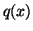, that estimates the underlying density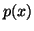, is known in addition to some constraints; then of all the densitieswhich satisfy the constraints we should select underlying probability density as that which yields the minimum relative information.

Given a prior density, we wish to arrive at a density function, when this underlying density functionsatisfies the usual probability constraint.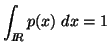(2.12)

and the partial informations in the form of averages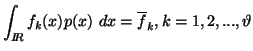(2.13)

By the minimum P.R.I.is to be such that it minimizes (2.9) subject to the constraints (2.10) and (2.11).

Using Lagrange's method of multipliers, we have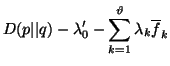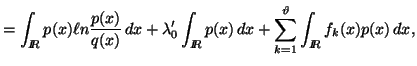(2.14)

where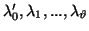are constants.

Equating the variations of this quantity with respect to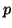to zero, we obtain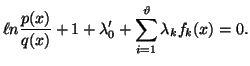(2.15)

This yields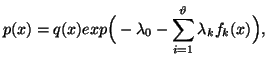(2.16)

where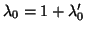. Thus we have

(i)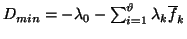.

(ii)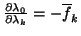.

Next we show thatobtained in (2.16) does indeed yield the M.R.I. and that it is unique.

The following property give some examples of the minimum relative information principle including the discrete case too.

Property 2.18. We have

(i) For the prior distribution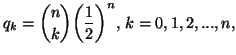under the constraint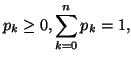the minimizing relative information distribution given by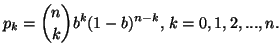is the Binomial distribution.
(ii) For the prior distribution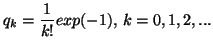under the constraints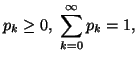and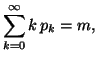the minimizing relative information distribution given by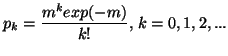is the Poisson distribution.
(iii)(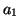) For the prior distribution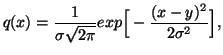under the constraints: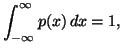and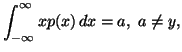the minimizing relative information distribution given by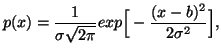is the normal distribution.
(iii)(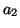) For the prior distributionunder the constraints:and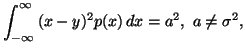the minimizing relative information distribution given by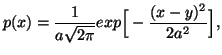is the normal distribution.
(iii)(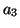) For the prior distributionunder the constraints: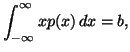and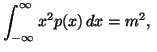where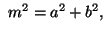the minimizing relative information distribution given by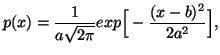is the normal distribution.
(iv)() For the prior distribution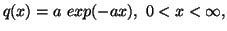under the constraints: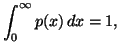and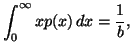the minimizing relative information distribution given by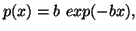is the exponential distribution.
(iv)() For the prior distributionunder the constraintsand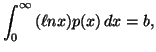the minimizing relative information distribution given by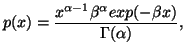is the gamma distribution.
(v)() For the prior distribution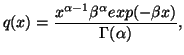under the constraintsandthe minimizing relative information distribution given by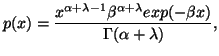is the gamma distribution.
(v)() For the prior distributionunder the constraintsandthe minimizing relative information distribution given by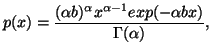is the gamma distribution.
(vi) For the prior distribution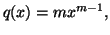under the constraintsand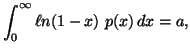the minimizing relative information distribution given by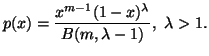is the Beta distribution.
(vii) For the prior distribution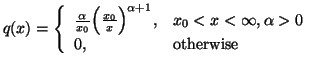under the constraints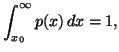and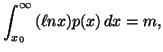the minimizing relative information distribution given by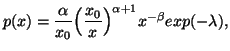is the Pareto distribution.

21-06-2001
Inder Jeet Taneja
Departamento de Matemática - UFSC
88.040-900 Florianópolis, SC - Brazil